# Introduction

## Welcome

Thank you for your interest in the incidentally package! The incidentally package is designed to generate random incidence matrices and bipartite graphs under different constraints or using different generative models.

The incidentally package can be cited as:

Neal, Z. P. (2022). incidentally: An R package to generate incidence matrices and bipartite graphs. OSF Preprints. https://doi.org/10.31219/osf.io/ectms

For additional resources on the incidentally package, please see https://www.rbackbone.net/. If you have questions about the incidentally package or would like an incidentally hex sticker, please contact the maintainer Zachary Neal by email (zpneal@msu.edu) or via Mastodon (@zpneal@mastodon.social). Please report bugs in the backbone package at https://github.com/zpneal/incidentally/issues.

## What are incidence matrices?

An incidence matrix is a binary $$r \times c$$ matrix I that records associations between $$r$$ objects represented by rows and $$c$$ objects represented by columns. In this matrix, $$I_{ij} = 1$$ if the ith row object is associated with the jth column object, and otherwise $$I_{ij} = 0$$. An incidence matrix can be used to represent a bipartite, two-mode, or affiliation network/graph, in which the rows represent one type of node, and the columns represent another type of node (e.g., people who author papers, species living in habitats) . An incidence matrix can also represent a hypergraph, in which each column represents a hyperedge and identifies the nodes that it connects.

For example: $I = \begin{bmatrix} 1 & 0 & 1 & 0 & 1\\ 0 & 1 & 1 & 1 & 1\\ 0 & 1 & 0 & 1 & 0 \end{bmatrix}$ is a $$3 \times 5$$ incidence matrix that represents the associations of the three row objects with the five column objects. If the rows represent people and the columns represent papers they wrote, then $$I_{1,1} = 1$$ indicates that person 1 wrote paper 1, while $$I_{1,2} = 0$$ indicates that person 1 did not write paper 2. One key property of an incidence matrix is its marginals, or when the matrix represents a bipartite graph, its degree sequences. In this example, the row marginals are $$R = \{3,4,2\}$$, and the column marginals are $$C = \{1,2,2,2,2\}$$. This incidence matrix can also be represented as a bipartite graph, where the row nodes are labeled with uppercase letters, and the column nodes are labeled with lowercase letters: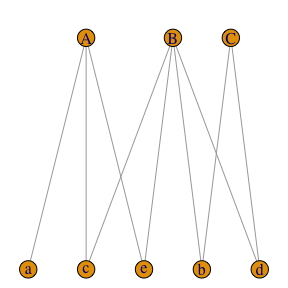The incidentally package can be loaded in the usual way:

set.seed(5)
library(incidentally)

Upon successful loading, a startup message will display that shows the version number, citation, ways to get help, and ways to contact me. Here, we also set.seed(5) to ensure that the examples below are reproducible.

## Package overview

The incidentally package offers multiple incidence matrix-generating functions that differ in how the resulting incidence matrix is constrained. These functions are described in detail below, but briefly:

# Fill and marginal constraints

## Fill/Density

The incidence.from.probability() function generates an incidence matrix or bipartite graph with a given probabaility $$p$$ that $$I_{ij} = 1$$, and thus an overall fill rate or density of approximately $$p$$. We can use it to generate a $$10 \times 10$$ incidence matrix in which $$Pr(I_{ij} = 1) \approx .2$$:

I <- incidence.from.probability(10, 10, .2)
I
#>       [,1] [,2] [,3] [,4] [,5] [,6] [,7] [,8] [,9] [,10]
#>  [1,]    0    0    1    0    0    0    0    1    0     0
#>  [2,]    0    0    0    0    0    1    0    1    0     0
#>  [3,]    0    0    0    0    0    0    1    1    0     0
#>  [4,]    0    0    0    0    0    0    0    0    1     1
#>  [5,]    1    1    0    1    1    0    0    0    0     0
#>  [6,]    0    0    0    1    0    0    1    0    0     0
#>  [7,]    0    0    0    0    0    0    0    0    1     0
#>  [8,]    0    0    0    0    0    0    0    0    0     1
#>  [9,]    0    0    0    0    0    0    0    1    0     0
#> [10,]    0    0    0    0    0    0    1    0    0     0
mean(I)  #Fill rate/density
#>  0.18

By default, incidence.from.probability() only generates incidence matrices in which no rows or columns are completely empty or full. We can relax this constraint, allowing some rows/columns to contain all 0s or all 1s by specifying constrain = FALSE:

I <- incidence.from.probability(10, 10, .2, constrain = FALSE)
I
#>       [,1] [,2] [,3] [,4] [,5] [,6] [,7] [,8] [,9] [,10]
#>  [1,]    0    0    0    1    0    0    0    0    0     0
#>  [2,]    0    0    0    1    0    0    1    0    0     0
#>  [3,]    0    0    0    0    0    0    0    1    0     1
#>  [4,]    0    0    0    0    1    0    0    1    0     0
#>  [5,]    0    0    1    0    0    0    0    0    0     1
#>  [6,]    0    0    0    0    0    0    1    0    1     0
#>  [7,]    0    0    0    0    1    0    1    1    0     0
#>  [8,]    0    0    0    0    1    0    0    0    0     0
#>  [9,]    0    0    0    0    1    0    0    0    0     0
#> [10,]    1    0    0    0    1    1    1    0    0     0
mean(I)  #Fill rate/Density
#>  0.2

## Marginal sums

The incidence.from.vector() function generates an incidence matrix with given row and column marginals, or a bipartite graph with given row and column node degrees. The generated matrix or graph represents a random draw from the space of all such matrices or graphs. We can use it to generate a random incidence matrix with $$R = \{3,4,2\}$$ and $$C = \{1,2,2,2,2\}$$:

I <- incidence.from.vector(c(4,3,2), c(1,2,2,2,2))
I
#>      [,1] [,2] [,3] [,4] [,5]
#> [1,]    1    1    1    0    1
#> [2,]    0    0    1    1    1
#> [3,]    0    1    0    1    0
rowSums(I)  #Row marginals
#>  4 3 2
colSums(I)  #Column marginals
#>  1 2 2 2 2

## Marginal distributions

The incidence.from.distributions() function generates an incidence matrix (or bipartite graph) in which the row and column marginals (or row and column node degrees) follow Beta distributions with given shape parameters. Beta distributions are used because they can flexibly capture many different distributional shapes:

A $$100 \times 100$$ incidence matrix with approximately uniformly distributed row and column marginals:

I <- incidence.from.distribution(R = 100, C = 100, P = 0.2,
rowdist = c(1,1), coldist = c(1,1))
#>
#> === Suggested manuscript text and citations ===
#> We used the incidentally package for R (v1.0.2; Neal, 2022) to generate a random incidence matrix with 100 rows whose sums are approximately distributed as B(1,1), and 100 columns whose sums are approximately distributed as B(1,1).
#>
#> Neal, Z. P. (2022). incidentally: An R package to generate incidence matrices and bipartite graphs. OSF Preprints. https://doi.org/10.31219/osf.io/ectms
hist(rowSums(I), main = "Row Marginals")
hist(colSums(I), main = "Column Marginals")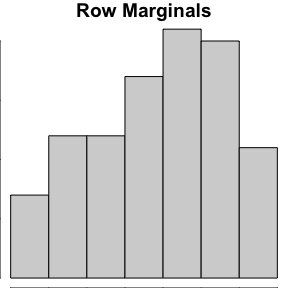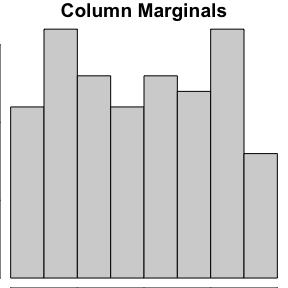A $$100 \times 100$$ incidence matrix with approximately right-tail distributed row and column marginals:

I <- incidence.from.distribution(R = 100, C = 100, P = 0.2,
rowdist = c(1,10), coldist = c(1,10))
#>
#> === Suggested manuscript text and citations ===
#> We used the incidentally package for R (v1.0.2; Neal, 2022) to generate a random incidence matrix with 100 rows whose sums are approximately distributed as B(1,10), and 100 columns whose sums are approximately distributed as B(1,10).
#>
#> Neal, Z. P. (2022). incidentally: An R package to generate incidence matrices and bipartite graphs. OSF Preprints. https://doi.org/10.31219/osf.io/ectms
hist(rowSums(I), main = "Row Marginals")
hist(colSums(I), main = "Column Marginals")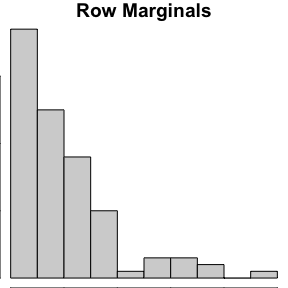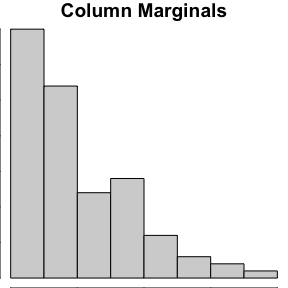A $$100 \times 100$$ incidence matrix with approximately left-tail distributed row and column marginals:

I <- incidence.from.distribution(R = 100, C = 100, P = 0.2,
rowdist = c(10,1), coldist = c(10,1))
#>
#> === Suggested manuscript text and citations ===
#> We used the incidentally package for R (v1.0.2; Neal, 2022) to generate a random incidence matrix with 100 rows whose sums are approximately distributed as B(10,1), and 100 columns whose sums are approximately distributed as B(10,1).
#>
#> Neal, Z. P. (2022). incidentally: An R package to generate incidence matrices and bipartite graphs. OSF Preprints. https://doi.org/10.31219/osf.io/ectms
hist(rowSums(I), main = "Row Marginals")
hist(colSums(I), main = "Column Marginals")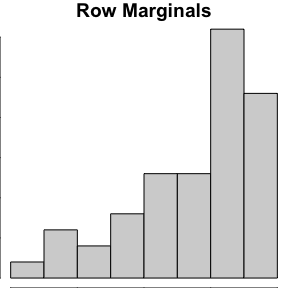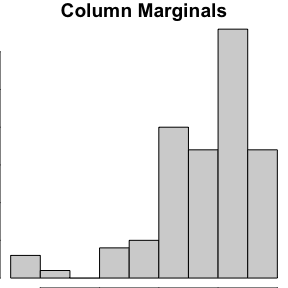A $$100 \times 100$$ incidence matrix with approximately normally distributed row and column marginals:

I <- incidence.from.distribution(R = 100, C = 100, P = 0.2,
rowdist = c(10,10), coldist = c(10,10))
#>
#> === Suggested manuscript text and citations ===
#> We used the incidentally package for R (v1.0.2; Neal, 2022) to generate a random incidence matrix with 100 rows whose sums are approximately distributed as B(10,10), and 100 columns whose sums are approximately distributed as B(10,10).
#>
#> Neal, Z. P. (2022). incidentally: An R package to generate incidence matrices and bipartite graphs. OSF Preprints. https://doi.org/10.31219/osf.io/ectms
hist(rowSums(I), main = "Row Marginals")
hist(colSums(I), main = "Column Marginals")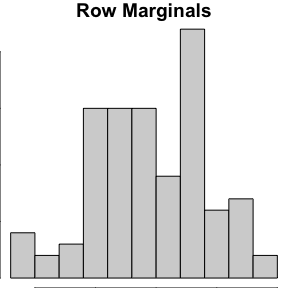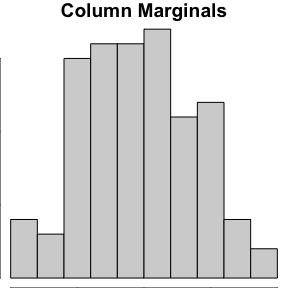A $$100 \times 100$$ incidence matrix with approximately constant row and column marginals:

I <- incidence.from.distribution(R = 100, C = 100, P = 0.2,
rowdist = c(10000,10000), coldist = c(10000,10000))
#>
#> === Suggested manuscript text and citations ===
#> We used the incidentally package for R (v1.0.2; Neal, 2022) to generate a random incidence matrix with 100 rows whose sums are approximately distributed as B(10000,10000), and 100 columns whose sums are approximately distributed as B(10000,10000).
#>
#> Neal, Z. P. (2022). incidentally: An R package to generate incidence matrices and bipartite graphs. OSF Preprints. https://doi.org/10.31219/osf.io/ectms
rowSums(I)
#>    20 20 20 20 20 20 20 20 20 20 20 20 20 20 20 20 20 20 20 20 20 20 20 20 20
#>   20 20 20 20 20 20 20 20 20 20 20 20 20 20 20 20 20 20 20 20 20 20 20 20 20
#>   20 20 20 20 20 20 20 20 20 20 20 20 20 20 20 20 20 20 20 20 20 20 20 20 20
#>   20 20 20 20 20 20 20 20 20 20 20 20 20 20 20 20 20 20 20 20 20 20 20 20 20
colSums(I)
#>    20 20 20 20 20 20 20 20 20 20 20 20 20 20 20 20 20 20 20 20 20 20 20 20 20
#>   20 20 20 20 20 20 20 20 20 20 20 20 20 20 20 20 20 20 20 20 20 20 20 20 20
#>   20 20 20 20 20 20 20 20 20 20 20 20 20 20 20 20 20 20 20 20 20 20 20 20 20
#>   20 20 20 20 20 20 20 20 20 20 20 20 20 20 20 20 20 20 20 20 20 20 20 20 20

Different types of Beta distributions can be combined. For example, we can generate a $$100 \times 100$$ incidence matrix in which the row marginals are approximately right-tailed, but the column marginals are approximately left-tailed:

I <- incidence.from.distribution(R = 100, C = 100, P = 0.2,
rowdist = c(1,10), coldist = c(10,1))
#>
#> === Suggested manuscript text and citations ===
#> We used the incidentally package for R (v1.0.2; Neal, 2022) to generate a random incidence matrix with 100 rows whose sums are approximately distributed as B(1,10), and 100 columns whose sums are approximately distributed as B(10,1).
#>
#> Neal, Z. P. (2022). incidentally: An R package to generate incidence matrices and bipartite graphs. OSF Preprints. https://doi.org/10.31219/osf.io/ectms
hist(rowSums(I), main = "Row Marginals")
hist(colSums(I), main = "Column Marginals")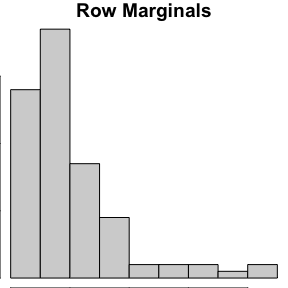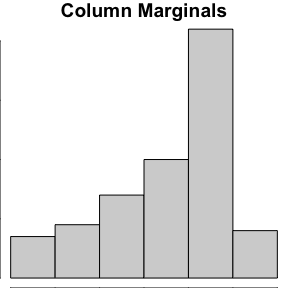# Generative models

Focus theory suggests that social networks form, in part, because individuals share foci such as activities that create opportunities for interaction . Individuals’ memberships in foci can be represented by an incidence matrix or bipartite graph. The social network that may emerge from these foci memberships can be obtained via bipartite projection, which yields an adjacency matrix or unipartite graph in which people are connected by shared foci .

Focus theory therefore explains how incidence/bipartite $$\rightarrow$$ adjacency/unipartite. However, it is also possible that individuals’ interactions in a social network can lead to the formation of new foci. That is, it is possible that adjacency/unipartite $$\rightarrow$$ incidence/bipartite. The incidence.from.adjacency() function implements three generative models (model = c("team", "group", "blau")) that reflect different ways that this might occur. These models are illustrated below, but are described in detail by Neal (2023).

## Teams model

The teams model mirrors a team formation process that depends on the structure of a given network in which cliques represent prior teams. Each row in the generated incidence matrix represents an agent in a given social network, while each column in the incidence matrix records the members of a team. Teams are formed from the incumbants of a randomly selected prior team (with probability $$p$$) and newcomers (with probability $$1-p$$).

Given an initial social network among 15 people, we can simulate their formation of one (k = 1) new team, where there is a p = 0.75 probability that a prior team member joins the the new team:

G <- erdos.renyi.game(15, .5)  #A random social network of 15 people, as igraph
I <- incidence.from.adjacency(G, k = 1, p = .75, model = "team")  #Teams model
#>
#> === Suggested manuscript text and citations ===
#> We used the incidentally package for R (v1.0.2; Neal, 2022a) to generate a random bipartite graph from a unipartite graph. The bipartite graph represents the memberships of the 15 nodes from the unipartite network in 1 newly-formed teams (Neal, 2022b).
#>
#> Neal, Z. P. (2022a). incidentally: An R package to generate incidence matrices and bipartite graphs. OSF Preprints. https://doi.org/10.31219/osf.io/ectms
#> Neal, Z. P. (2022b). The Duality of Networks and Foci: Generative Models of Two-Mode Networks from One-Mode Networks. arXiv:2204.13670 [cs.SI]. https://doi.org/10.48550/arXiv.2204.13670
class(I)  #Incidence matrix returned as igraph object
#>  "igraph"
V(I)$shape <- ifelse(V(I)$type, "square", "circle")  #Add shapes
plot(G, main="Prior Team Collaborations")
plot(I, layout = layout_as_bipartite(I), main="New Team")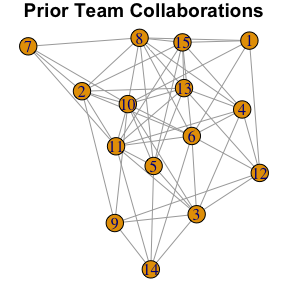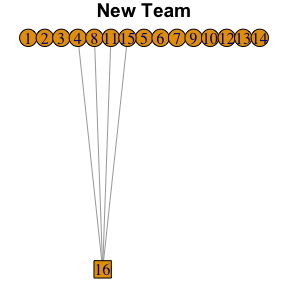Notice that because the social network G is supplied as a igraph object, the generated object I is returned as an igraph bipartite network, which facilitates subsequent plotting and analysis. In this example, a new team (node 16) is formed by four agents (nodes 4, 8, 11, and 15). The function simulates the formation of the team invisibly, so we cannot see exactly why this particular team formed. This team may have emerged from the prior 4-member team of 4, 8, 13, and 15 (they are a clique in the social network), where three positions on the new team are filled by incumbents (4, 8, and 15), while the final position is filled by a newcomer (11).

## Clubs model

The clubs model mirrors a social club formation process in which current club members try to recruit their friends. To ensure a minimum level of group cohesion, potential recruits join a newly-forming club only if doing so would yield a club in which the members’ social ties have a density of at least $$p$$. Each row in the generated incidence matrix represents an agent in a given social network, while each column in the incidence matrix records the members of a club.

Given an initial social network among 15 people, we can simulate their formation of one (k = 1) new club, where the club has a minimum density of p = 0.75:

G <- erdos.renyi.game(15, .33)  #A random social network of 15 people, as igraph
I <- incidence.from.adjacency(G, k = 1, p = .75, model = "club")  #Groups model
#>
#> === Suggested manuscript text and citations ===
#> We used the incidentally package for R (v1.0.2; Neal, 2022a) to generate a random bipartite graph from a unipartite graph. The bipartite graph represents the memberships of the 15 nodes from the unipartite network in 1 newly-formed club (Neal, 2022b).
#>
#> Neal, Z. P. (2022a). incidentally: An R package to generate incidence matrices and bipartite graphs. OSF Preprints. https://doi.org/10.31219/osf.io/ectms
#> Neal, Z. P. (2022b). The Duality of Networks and Foci: Generative Models of Two-Mode Networks from One-Mode Networks. arXiv:2204.13670 [cs.SI]. https://doi.org/10.48550/arXiv.2204.13670
V(I)$shape <- ifelse(V(I)$type, "square", "circle")  #Add shapes
plot(G, main="Social Network")
plot(I, layout = layout_as_bipartite(I), main="New Group")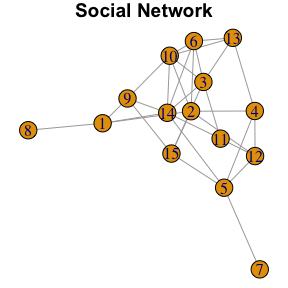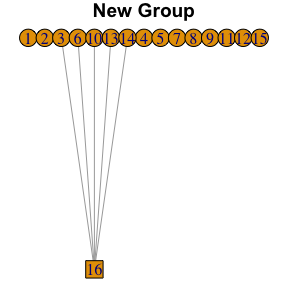In this example, a new club (node 16) is joined by five agents (nodes 3, 6, 10, 13, and 14). The social network among these group members is very cohesive (density = .9). The members of this group may have attempted to recruit other friends, but these others did not join because doing so would have reduced the group’s cohesion. For example, agent 10 may have tried to recruit agent 9. However, if agent 9 had joined the club, the new club would have a density of 0.73, which is lower than the minimum threshold. Therefore, agent 9 did not join the club.

## Organizations model

The Organizations model mirrors an organizational recruitment process . The given social network is embedded in a $$d$$ dimensional social space in which the dimensions are assumed to represent meaningful social distinctions, such that socially similar people are positioned nearby. Organizations recruit members from this space, recruiting people inside their niche with probability $$p$$, and outside their niche with probability $$1-p$$. Each row in the generated incidence matrix represents an agent in a given social network, while each column in the incidence matrix records the members of an organization.

Given a social network among 15 people, we can simulate their recruitment by one (k = 1) new organization, where there is a p = 0.95 probability that an individual inside an organization’s niche becomes a member:

G <- erdos.renyi.game(15, .5)  #A random social network of 15 people, as igraph
I <- incidence.from.adjacency(G, k = 1, p = .95, model = "org")  #Groups model
#>
#> === Suggested manuscript text and citations ===
#> We used the incidentally package for R (v1.0.2; Neal, 2022a) to generate a random bipartite graph from a unipartite graph. The bipartite graph represents the memberships of the 15 nodes from the unipartite network in 1 newly-formed organizations (Neal, 2022b).
#>
#> Neal, Z. P. (2022a). incidentally: An R package to generate incidence matrices and bipartite graphs. OSF Preprints. https://doi.org/10.31219/osf.io/ectms
#> Neal, Z. P. (2022b). The Duality of Networks and Foci: Generative Models of Two-Mode Networks from One-Mode Networks. arXiv:2204.13670 [cs.SI]. https://doi.org/10.48550/arXiv.2204.13670
V(I)$shape <- ifelse(V(I)$type, "square", "circle")  #Add shapes
plot(G, layout = layout_with_mds(G), main="Social Network")
plot(I, layout = layout_as_bipartite(I), main="New Organizations")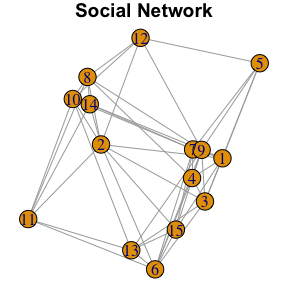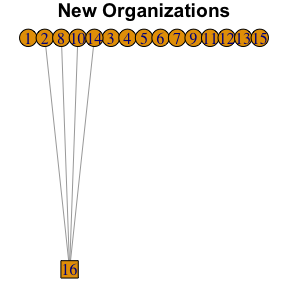The social network is plotted using a Multidimensional Scaling layout, and therefore shows the nodes’ positions in the abstract Blau Space from which organizations recruit members. In this example, a new organization (node 16) recruits four members (nodes 2, 8, 10, and 14). This organization’s niche is located on the left side of the space, where it very successfully (because p = 0.95) recruits all the people in this region.

# Utilities

## Randomization

The curveball() function uses the curveball algorithm to randomize an incidence matrix or bipartite graph while preserving its marginal/degree sequence. The result is uniformly randomly sampled from the space of all incidence matrices (bipartite graphs) with the give marginal (degree) sequences.

For example, given a $$3 \times 3$$ matrix with row marginals $$R = \{2,1,1\}$$ and column marginals $$C = \{1,1,2\}$$, we can randomly sample a new matrix with the same row and column marginals:

I <- matrix(c(1,0,0,0,1,0,1,0,1),3,3)
I
#>      [,1] [,2] [,3]
#> [1,]    1    0    1
#> [2,]    0    1    0
#> [3,]    0    0    1
curveball(I)
#>      [,1] [,2] [,3]
#> [1,]    1    1    0
#> [2,]    0    0    1
#> [3,]    0    0    1

## Block models

The add.blocks() function shuffles an incidence matrix or bipartite graph to have a block structure or planted partition while preserving the row and column marginals/degrees. For example, after generating a 10 $$\times$$ 10 incidence matrix with a density of .2, we can plant a partition in which the within-group density = 0.75. In this example, the first 5 row nodes and first 5 column nodes are assigned to block 1, while the second 5 row nodes and second 5 column nodes are assigned to block 2:

I <- incidence.from.probability(R = 10, C = 10, P = .2)
blocked <- add.blocks(I, density = .75,
rowblock = c(1,1,1,1,1,2,2,2,2,2),
colblock = c(1,1,1,1,1,2,2,2,2,2))
I  #Original matrix
#>       [,1] [,2] [,3] [,4] [,5] [,6] [,7] [,8] [,9] [,10]
#>  [1,]    0    0    1    1    1    0    0    0    0     0
#>  [2,]    1    1    0    0    0    1    1    0    0     0
#>  [3,]    1    0    0    0    0    1    0    1    0     1
#>  [4,]    0    0    0    1    0    0    1    0    1     1
#>  [5,]    0    0    1    0    0    0    1    0    0     0
#>  [6,]    0    0    1    1    0    0    0    1    0     0
#>  [7,]    0    0    0    0    1    0    0    0    0     0
#>  [8,]    0    1    1    0    0    0    0    1    1     0
#>  [9,]    0    0    0    0    0    1    0    0    0     0
#> [10,]    0    0    0    0    1    1    0    0    0     0
blocked  #Blocked matrix
#>       [,1] [,2] [,3] [,4] [,5] [,6] [,7] [,8] [,9] [,10]
#>  [1,]    0    0    1    1    1    0    0    0    0     0
#>  [2,]    1    1    1    0    0    1    0    0    0     0
#>  [3,]    1    1    1    0    1    0    0    0    0     0
#>  [4,]    0    0    0    1    1    0    1    0    0     1
#>  [5,]    0    0    1    0    0    0    1    0    0     0
#>  [6,]    0    0    0    1    0    0    1    1    0     0
#>  [7,]    0    0    0    0    0    0    0    0    1     0
#>  [8,]    0    0    0    0    0    1    0    1    1     1
#>  [9,]    0    0    0    0    0    1    0    0    0     0
#> [10,]    0    0    0    0    0    1    0    1    0     0
all(rowSums(I)==rowSums(blocked)) #Row marginals preserved
#>  TRUE
all(colSums(I)==colSums(blocked)) #Column marginals preserved
#>  TRUE